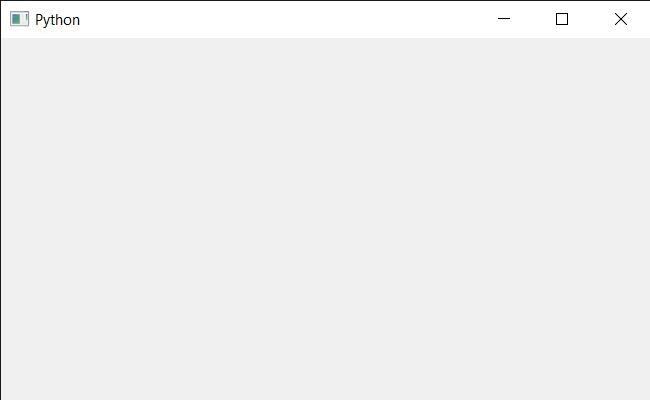# PyQt5 QCalendarWidget – Setting Close Event

• Last Updated : 27 Jan, 2022

In this article we will see how we can implement the close event for the QCalendarWidget. This event handler is called with the given event when Qt receives a window close request for a calendar from the window system. By default, the event is accepted and the calendar is closed. We can reimplement this function to change the way the widget responds to window close requests.

Below is the Calendar class code

```# QCalendarWidget Class
class Calendar(QCalendarWidget):

# constructor
def __init__(self, parent=None):
super(Calendar, self).__init__(parent)

# overriding the closeEvent method
def closeEvent(self, event):

# setting text to the label
print("Close Event Called")

```

Implementation steps:
1. Create a Calendar class that inherits the QCalendarWidget
2. Inside the Calendar class override the closeEvent and inside the event print the text
3. Create a main window class
4. Create a Calendar object inside the main window
5. Set various properties to the calendar
6. Close the calendar

Below is the implementation

## Python3

 `# importing libraries ` `from` `PyQt5.QtWidgets ``import` `*`  `from` `PyQt5 ``import` `QtCore, QtGui ` `from` `PyQt5.QtGui ``import` `*`  `from` `PyQt5.QtCore ``import` `*`  `import` `sys ` ` `  `# QCalendarWidget Class ` `class` `Calendar(QCalendarWidget): ` ` `  `    ``# constructor ` `    ``def` `__init__(``self``, parent ``=` `None``): ` `        ``super``(Calendar, ``self``).__init__(parent) ` ` `  `    ``# overriding the closeEvent method ` `    ``def` `closeEvent(``self``, event): ` ` `  `        ``# setting text to the label ` `        ``print``(``"Close Event Called"``) ` ` `  ` `  `class` `Window(QMainWindow): ` ` `  `    ``def` `__init__(``self``): ` `        ``super``().__init__() ` ` `  `        ``# setting title ` `        ``self``.setWindowTitle(``"Python "``) ` ` `  `        ``# setting geometry ` `        ``self``.setGeometry(``100``, ``100``, ``650``, ``400``) ` ` `  `        ``# calling method ` `        ``self``.UiComponents() ` ` `  `        ``# showing all the widgets ` `        ``self``.show() ` ` `  `    ``# method for components ` `    ``def` `UiComponents(``self``): ` ` `  `        ``# creating a QCalendarWidget object ` `        ``# as Calendar class inherits QCalendarWidget ` `        ``self``.calendar ``=` `Calendar(``self``) ` ` `  `        ``# setting geometry to the calendar ` `        ``self``.calendar.setGeometry(``50``, ``10``, ``400``, ``250``) ` ` `  `        ``# setting cursor ` `        ``self``.calendar.setCursor(Qt.PointingHandCursor) ` ` `  `        ``# close the calendar ` `        ``self``.calendar.close() ` ` `  ` `  ` `  ` `  `# create pyqt5 app ` `App ``=` `QApplication(sys.argv) ` ` `  `# create the instance of our Window ` `window ``=` `Window() ` ` `  `# start the app ` `sys.exit(App.``exec``()) `

Output :

`Close Event Called`My Personal Notes arrow_drop_up
Recommended Articles
Page :